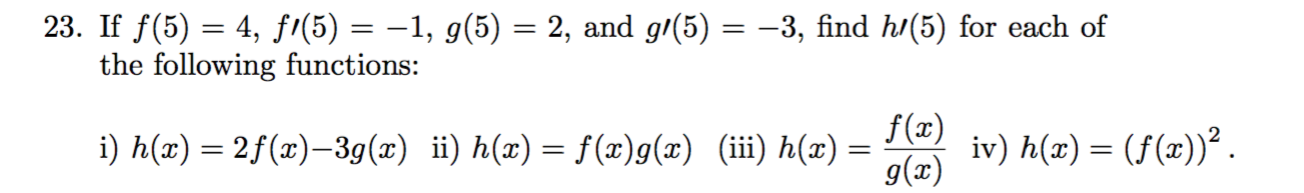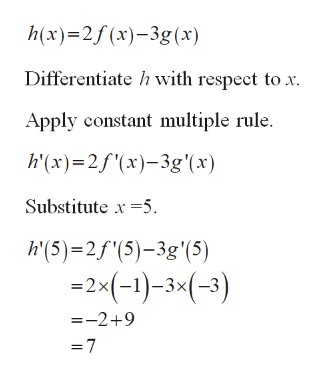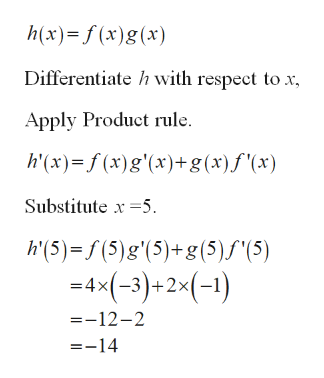# 23. If f(5) = 4, fi(5) = –1, g(5) = 2, and g/(5) = -3, find h/(5) for each ofthe following functions:f(x)iv) h(x) = (f(x))².g(x)i) h(x) = 2f(x)–3g(x) ii) h(x) = f(x)g(x) (iii) h(x) =

Question
2 viewshelp_outlineImage Transcriptionclose23. If f(5) = 4, fi(5) = –1, g(5) = 2, and g/(5) = -3, find h/(5) for each of the following functions: f(x) iv) h(x) = (f(x))². g(x) i) h(x) = 2f(x)–3g(x) ii) h(x) = f(x)g(x) (iii) h(x) = fullscreen
check_circle

Step 1

Since you have posted a question with multiple sub-parts, we will solve first three sub-parts for you. To get remaining sub-part solved please repost the complete question and mention the sub-parts to be solved.

Step 2

Part (i):help_outlineImage Transcriptioncloseh(x)=2f(x)-3g (x) Differentiate h with respect to x. Apply constant multiple rule. h'(x)=2f'(x)-3g'(x) Substitute x =5. h'(5)=2f"(5)-3g'(5) =2x(-1)-3×(-3) =-2+9 =7 fullscreen
Step 3

Part (ii):

...help_outlineImage Transcriptioncloseh(x)=f (x)g(x) Differentiate h with respect to x, Apply Product rule. h'(x)= f (x)g'(x)+g(x)f'(x) Substitute x =5. h'(5) = f (5)g'(5)+g(5)S"(5) =4x(-3)+2×(-1) =-12-2 =-14 fullscreen

### Want to see the full answer?

See Solution

#### Want to see this answer and more?

Solutions are written by subject experts who are available 24/7. Questions are typically answered within 1 hour.*

See Solution
*Response times may vary by subject and question.
Tagged in

### Calculus